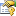﻿ BaseLeastSquaresMethod Properties# BaseLeastSquaresMethod Properties

The BaseLeastSquaresMethod type exposes the following members.Properties
NameDescriptionConvergence
Gets or sets the convergence verification method.CurrentIteration
Gets the current iteration number.Function
Gets or sets a parameterized model function mapping input vectors into output values, whose optimum parameters must be found.Gradient
Gets or sets a function that computes the gradient vector in respect to the function parameters, given a set of input and output values.HasConverged
Gets whether the algorithm has converged.Iterations Obsolete.MaxIterations
Gets or sets the maximum number of iterations performed by the iterative algorithm. Default is 100.NumberOfParameters
Gets the number of variables (free parameters) in the optimization problem.NumberOfVariables Obsolete.
Gets the number of variables (free parameters) in the optimization problem.ParallelOptions
Gets or sets the parallelization options for this algorithm.
(Inherited from ParallelLearningBase.)Solution
Gets the solution found, the values of the parameters which optimizes the function, in a least squares sense.Token
Gets or sets a cancellation token that can be used to cancel the algorithm while it is running.
(Inherited from ParallelLearningBase.)Tolerance
Gets or sets the maximum relative change in the watched value after an iteration of the algorithm used to detect convergence. Default is zero.Value
Gets the value at the solution found. This should be the minimum value found for the objective function.
TopSee Also